# How to Differentiate between Analog and Digital Computers

## Analog Computers

We have heard a lot about digital computers, but, analog computers are rarely mentioned. Not because they don’t exist, but because people think computers are digital only. We come across so many analog computers, but we hardly notice them as a computer. The basic difference between analog and digital computers is the type of data they process. Analog computers process analog, i.e. continuously varying, data. Digital computer process data which is binary, i.e. in the form of 0 and 1.

Analog computers operate on mathematical variables in the form of physical quantities that are continuously varying. For example temperature, pressure, voltages, etc. The basis of the working of the analog computers is modeling. Any real physical process can always be replaced by a mathematical model having the same properties. Control Engineers often convert a mechanical or hydraulic system to its electrical analogy to make a mathematical model for computation. This analogy or conversion of a physical process like a suspended mass in a spring to its electrical model and consequently the mathematical model is the basis of analog computing. After the modeling has been done the computation becomes very easy and convenient.

The advantages of analog computers are that they show the solutions in a simple and graphical manner in little time. In a digital computer the real time changes require complex programming and graphical programs. In an analog computer the output can be connected to an oscilloscope and results can be seen.

The disadvantages of analog computers are that they are not versatile and they are not very accurate. The accuracy of the analog computers is rather limited and dependent on a number for factors like spread in circuit parameters, inaccurate assembly, wiring problems, external influences like magnetic field, changes in ambient temperature and pressure, etc. To obtain accuracy in analog computers before a computation all op-amps must be checked for DC drift.

Famous examples of analog computers are the Planimeter, the nomogram, operational amplifiers, mechanical integrators, slide rules, tide predictors, electric integrators that solve partial differential equations, electronic machines that solve ordinary differential equations, machines to solve algebraic equations, the Norden bomb sight, and neural networks.

## Analog Computer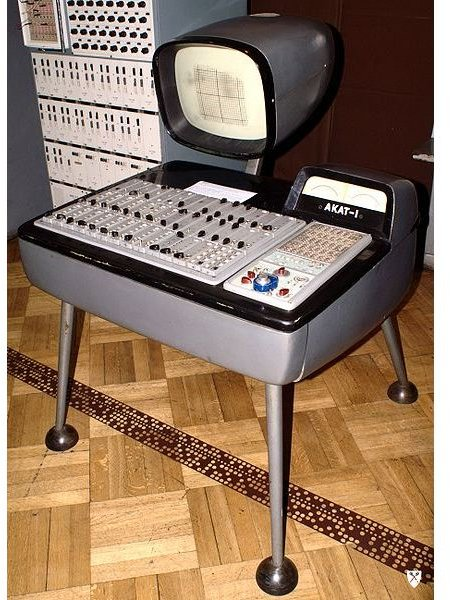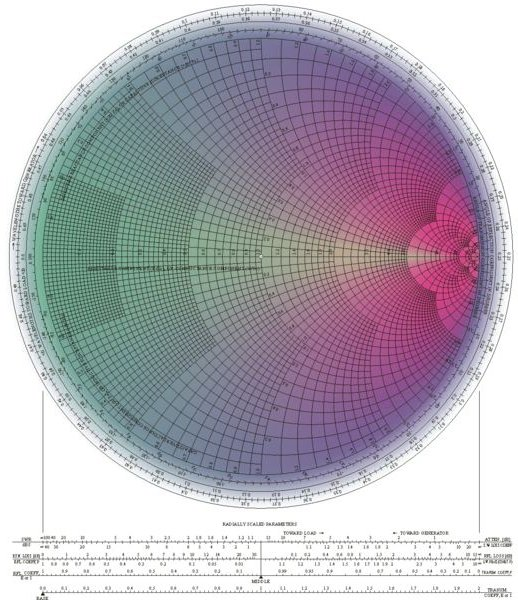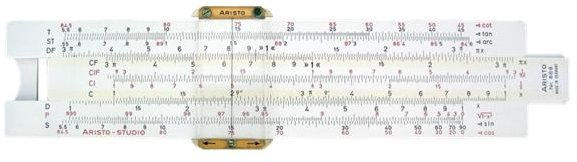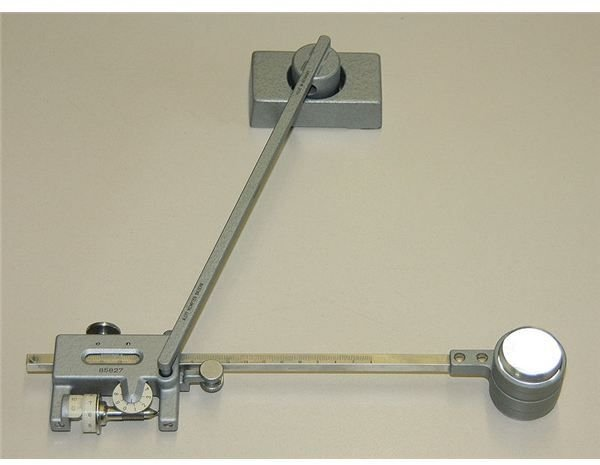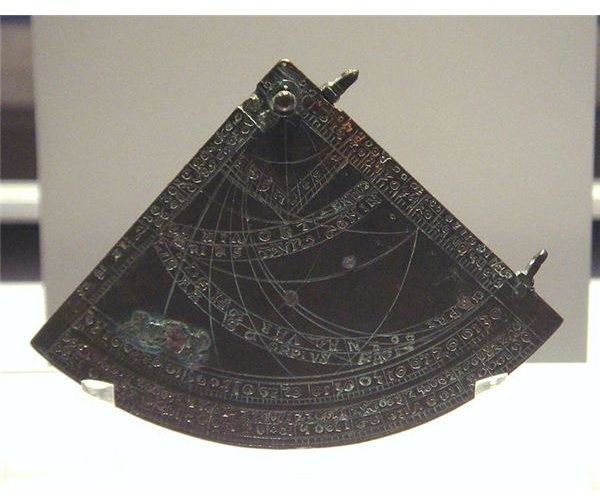## Digital Computers

Digital computers deal with mathematical variables in form of numbers that represent discrete values of physical quantities. The advantages of digital computers are that they are versatile, reprogrammable, accurate, and less affected by outside disturbances. In contrast to analog computers, digital machines work on numbers. Each variable is converted into numbers and each number into binary form, i.e. 0 and 1. It is this combination of 0 and 1 that does all the calculations. All modern computers, laptops, and calculators are all digital computers.

## Digital Computing Devices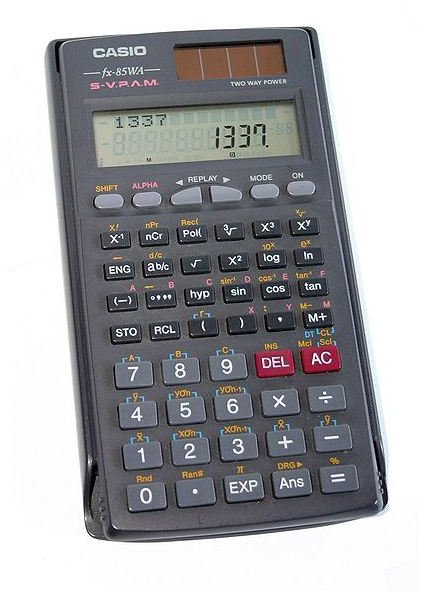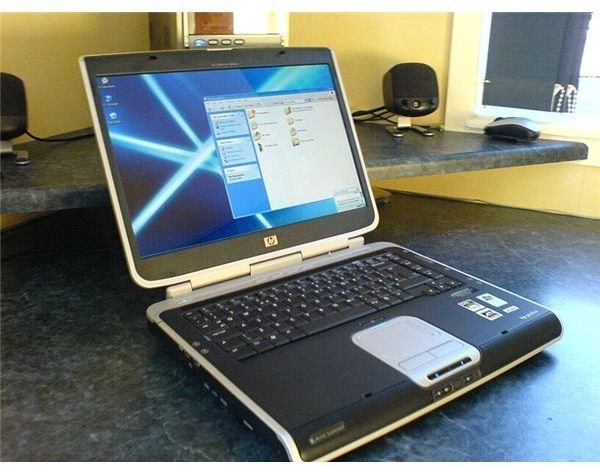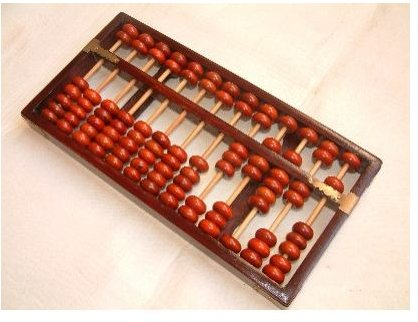## References

Digital Computer Fundamentals by Thomas C. Bartee

Chipping In by Br. Eric S. D’Souza

An Introduction to Computers by N. Sergeev, N. Vashkevich

Analog Circuits & Digital Fundamentals: by Dr. A.K. Sharma

IBM Antique Attic Volume One, Two, and Three

## Image Credits

Analog Computer: Wikimedia Commons

Nomogram: Wikimedia Commons

Slide Rule: Wikimedia Commons

Planimeter: Wikimedia Commons

Astrolabe: Wikimedia Commons

Calculator: Wikimedia Commons

Laptop: Wikimedia Commons

Abacus: Wikimedia Commons